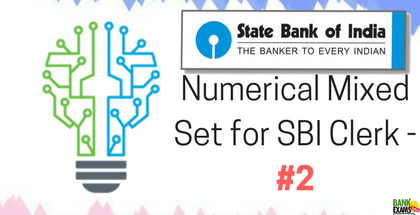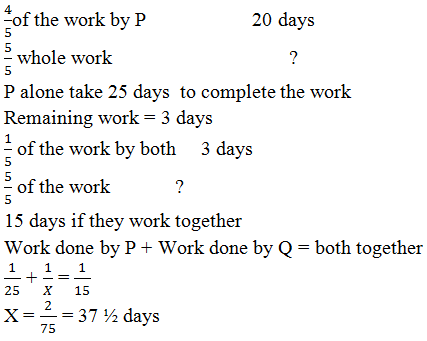# Numerical Mixed Set for SBI Clerk - Part 2## Question 1.

On a certain sum of money at the simple rate of interest for the 6 ¼ years becomes 3/8 of the principal amount. Fins the rate of interest.
a) 6.5%
b) 6%
c) 4%
d) 5%
e) 9%

## Question 2.

Capacities of three vessels are 2 litres, 3 litres and 5 litres. The contribution of milk in these three vessels is 90%, 70%, and 80% respectively and it is mixed in the third vessel. Find the ratio of milk to water.
a) 79:21
b) 73:22
c) 69:11
d) 53:41
e) 42:23

## Question 3.

A Woman bought chair and sold it and gain 10% of cost price. If she had bought it at 20% less and if for Rs 10 more, she would have gain 40%. Find the cost price.
a) 450
b) 525
c) 500
d) 621
e) 650

## Question 4.

Divide Rs 20816 in such a way that compound interest on 1st part of 7 year is same to the 2nd part of 9 year at 4% rate of interest per annum for both years.
a) 10816, 10000
b) 12324, 8492
c) 14652,6164
d) 11655, 9161
e) 13540, 7276

## Question 5.

P can do 4/5 of the work in 20 days. He then calls Q and both together finish the remaining work in 3 day. How long Q would take to do the whole work.
a) 32 days
b) 27 days
c) 29 days
d) 37 1/ 2 days
e) 35 ¼ days

## Question 6.

Two trains are 150 meter and 120 meter long moving from opposite direction, cross each other in 10 seconds if the speed of the 2nd train is 43.2 km/ph. finds the speed of second train?
a) 49 km/ph
b) 54 km/ph
c) 59 km/ph
d) 64 km/ph
e) 67 km/ph

## Question 7.

8 taps are filled a tank, some are inlet and some are outlet pipes. Each inlet pipe can fill the tank in 12 hours and outlet pipe can empty the tank in36 hours. Then, calculate the no. of inlet pipes, if the whole tank get filled in 3 hour.
a) 5
b) 1
c) 9
d) 2
e) 7

## Question 8.

A and B are two partners with capital 50000 and 70000 and agreed that 70% of the profit should be divided equally between then and the remaining profit in the ration of their capital. If one partner gets Rs 90 more than the other. Find total profit?
a) 1500
b) 1800
c) 1250
d) 1950
e) 1457

## Question 9.

A man can row at 5 km/h in still water. If the river is running at 1 km/h, it takes him 75 minutes to row to a place and back. How far is the place?
a) 3.75 km
b) 3 km
c) 7.5 km
d) 4.25 km
e) 2 km

## Question 10.

2 4 3 9 4 61 5 52 6 63 7 ?
a) 52
b) 29
c) 35
d) 94
e) 25

## Solutions

### Ans 2. (79:21)

Quantity in litre [Milk water]
2                          90%   10%
3                          70%   30%
5                          80%   20%

2×9=18 2×1=2
3×7=21 3×3=9
5×8=40 5×2=10
18+21+40 = 79
2+9+1 = 21
79:21

### Ans 3. (500 )

Cost price selling price Initial cost price = 100
10% on 100 = 110 s.p.
20% less on cost price of Rs 100 = 80
40% on Rs 80 = Rs112 selling price
Difference = 112 – 100 = 2
Rs 2           Rs 10
Rs 100 ?
500

### Ans 4. (10816, 10000 )

1st part                       2nd part
9 year (7 year + 2 year) 7 year
So interest on 7 year is same for both
4% interest on 2 year at C.I. = 8.16
1st part = 100 + 8.16 = 108.16
2nd part = 100
Total= 108.16 + 100 = 208.16
208.16           20816
108.16 ?
10816
208.16           20816
100 ?
10000

### Ans 5. (37 ½ days)### Ans 6.  (54 km/hr )### Ans 7. (5)

1 day work
Inlet pipe    12 +3
Outlet pipe 36 -1
By taking L.C.M. of 12 and 36 +3 and -1 came.
36 hours/3 hour = 12 day work of 8 pipes together
If all 8 pipes can inlet = 8 ×3 = 24
If all 8 pipes can outlet = 8 × -1 = -8
Solve it by allegation method
Work of inlet pipe work of outlet pipe
24                     -8
12
20                    12
Ratio of 20:15 = 5:3
Inlet pipe = 5
Outlet pipe = 3

### Ans 8. (1800)

Total profit = 100
70% of 100 = 70
30% of 100 = 30
A = 50000
B = 70000
Ratio of it 5:7 = 12
So Divide Rs 70 between them equally = 70
A = Rs 35
B = Rs 35
Divide Rs 30 in ratio of 5:7
A = 12.5
B= 17.5
Difference of it = Rs 5
Difference of R5       Rs 90
Rs 100      ?
RS 1800

### Ans 9. (3Km)### Ans 10. (94)

every second number is reverse of the square of a number
2 = 4
3 = 9
4 = 61 (Reverse of 16)
5 = 52 (Reverse of 25)
6 = 63 (Reverse of 36)
7 = 94 (Reverse of 49)